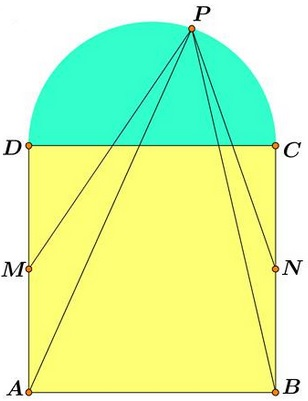Semicircle on Square

Introduction and acknowledgment

Ruben Dario and Miguel Ochoa Sanchez (Peru) have posted an elegant problem on top of a beautiful picture:The solution below is due to Leo Giugiuc (Romania).

Problem

$P$ is a point on the semicircle with diameter $CD$ constructed on a side of the square $ABCD.$ $M$ is the midpoint of $AD,$ $N$ the midpoint of $CD.$$\displaystyle\frac{AP^{4}-BP^{4}}{MP^{4}-NP^{4}}=2.$

Solution

Solution makes a skillful use of Cartesian coordinates. Choose the coordinates so that the origin is at the midpoint of $CD,$ $C=(-1,0),$ $D=(1,0),$ $A=(-1,-2),$ $B=(1,-2),$ $P=(\cos t, \sin t).$ Then, $M=(-1,-1)$ and $N=(1,-1).$ Further,

\begin{align} AP^{2} &= (-1-\cos t)^{2}+(-2-\sin t)^{2}=6+2\cos t+4\sin t,\\ BP^{2} &= (1-\cos t)^{2}+(-2-\sin t)^{2}=6-2\cos t+4\sin t,\\ MP^{2} &= (-1-\cos t)^{2}+(-1-\sin t)^{2}=3+2\cos t+2\sin t,\\ NP^{2} &= (1-\cos t)^{2}+(-1-\sin t)^{2}=3-2\cos t+2\sin t. \end{align}

Now, instead of squaring these expressions in order to obtain the fourth powers, Leo cleverly uses the identity $a^{4}-b^{4}=(a^{2}+b^{2})(a^{2}-b^{2})$ so that

\begin{align} AP^{4}-BP^{4} &= (12 + 8\sin t)\cdot 4\cos t,\\ MP^{4}-NP^{4} &= (6 +4\sin t)\cdot 4\cos t, \end{align}

which proves the statement, regardless of the value of $t,$ implying that the statement is true also when $P$ lies on the complete circle with diameter $CD.$

As Pat Ballew has observed, the points corresponding to $\displaystyle t=\pm\frac{\pi}{2}$ require special consideration. There are at least two ways to handle the situation. One is to use limits, the other is to repeated the calculations specifically for those points. This is left as an exercise to the reader.

This problem led to more general questions and some answers.